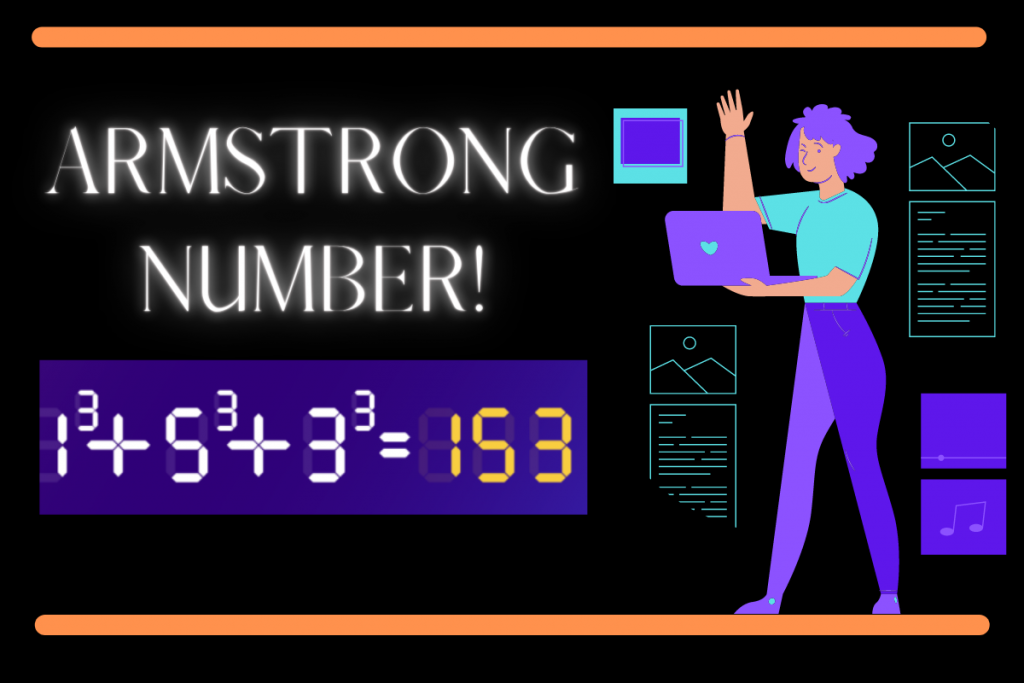# Armstrong Number in Python – Easy ImplementationHello there! Today let us learn something Interesting, Armstrong Number. We would be understanding what the number is and then implement a program to check if a number is an Armstrong Number or not.

## What is an Armstrong Number?

A number of `n` digits is an Armstrong number, if sum of each digit raised to the power of the no of digits is equal to the original number.

Armstrong Number definition is : abcd…(n-digits) = a^n + b^n + c^n + d^n + . . . . . and so on.

## Examples of Armstrong Number

### Example 1 : 153

Total number of digits = 3

Calculation (digit – wise ) = 1^3 + 5^3 + 3^3 = 1 + 125 + 27 = 153

The calculation done is directly equal to the original number. Hence the number is an Armstrong number.

### Example 2 : 548834

Total number of digits = 6

Calculation (digit – wise) = 5^6 + 4^6 +8^6 + 8^6 + 3^6 + 4^6 = 15625 + 4096 + 262144 + 262144 + 729 + 4096 = 548834

The calculations done is directly equal to the original number. Hence the number is an Armstrong Number.

## Algorithm to check Armstrong Number

To check if a number is an Armstrong number, one needs to follow the following steps

1. Count the number of digits in the number.
2. Each digit is accessed one after another with the help of mod and division operations
3. Each digit is raised to the power of the number of digits and the result is stored in a separate variable
4. Steps 2 and 3 are repeated until the digits exhaust.
5. Check the result calculated with the original number
• If It matches: Armstrong Number
• Otherwise: Not an Armstrong Number

## PseudoCode for Armstrong Number

The code below shows the pseudo code to check if a number is an Armstrong Number:

```READ n
CALCULATE NO OF DIGITS n_digit
MAKE A COPY OF n
result=0

CHECK DIGIT BY DIGIT:
WHILE n!=0
GET CURRENT DIGIT : digit = n % 10
UPDATE RESULT : result = result + digit^(n_digit)
TRIM THE LAST DIGIT : n = n / 10
ENDWHILE

CHECK FOR ARMSTRONG NUMBER:
IF result==COPY OF n
PRINT "ARMSTRONG NUMBER"
ELSE
PRINT "NOT AN ARMSTRONG NUMBER"
```

## Implementing Armstrong Number Checking in Python

Now that we know about what Armstrong Number is and the steps to implement that, let’s implement the Armstrong checking line by line.

### 1. Create the intital variables

We first take an input `n` and then calculate the length of the input. We also store a copy of the input so that no matter how much we change the original number, we have the copy to check Armstrong’s number later. We also initialized the result as 0.

The code for the same is shown below:

```n = input()
n_digit = len(n)
n=int(n)
copy_n=n
result = 0
```

### 2. Traversing through the number and Updating Result

To access each digit we take the modulus of the number ( mod 10 ) to extract the last digit of the number. The next step involves updating the result as the sum of the previous result and the digit raised to the power of the number of digits.

The last and final step we take is divide the number by 10 to drop the last digit from the number. The same process is repeated until there are no more digits are left in the number.

The code for the same is shown below:

```while(n!=0):
digit = n%10
result=result+pow(digit,n_digit)
n=int(n/10)
```

### 3. Checking if the number is an Armstrong Number or not

The final step is to check the copy of the number we created earlier with the result calculated to finally tell if the number is an Armstrong number or not. The code for the same is shown below:

```if(result==copy_n):
print("Armstrong Number!")
else:
print("Not an Armstrong Number!")
```

## Output Samples for the code

For now I tested the program for four inputs. The outputs for all four is shown below:

### Number 1: 153

```153
Armstrong Number!
```

### Number 2: 121

```121
Not an Armstrong Number!
```

### Number 3: 548834

```548834
Armstrong Number!
```

### Number 4: 9468632

```9468632
Not an Armstrong Number!
```

## Conclusion

Congratulations! You have successfully learned about Armstrong Number and implemented the same!

But don’t stop here! Keep Reading and Learning!